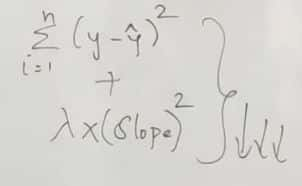# Lasso And Ridge RegularizationAmal Aj Jan 06 2021 · 2 min read

This requires a prior knowledge of Linear Regression. Go check my last blog about Linear Regression by clicking here.

OK, Let's start by understanding what is Regularization?

In layman term, Regularization helps the over-fitting problem of Linear Regression by shrinking the corresponding coefficients towards zero .

By this way, it discourages learning a more complex model as to avoid over-fitting. Over-fitting is a error due to which the model is trying too hard to capture noise and fit in the data .

In linear regression  , Y= mx+c; the entire relation will depend on m&c.

• In Regularization, we need a penalty term to the linear equation so it will also contribute and help you find better relations in the model.
• Regularization restricts the coefficient of features in which a small change in m results a large difference in Y so as to avoid over-fitting.
• Lets get into main agenda of this blog,Lasso & Ridge are the Regularization Techniques used.

### 1) Lasso Regression(L1 Regularization)

LASSO(Least Absolute Shrinkage and Selection Operator)

Here observe the keywords- ABSOLUTE , SELECTION (because this is what lasso does!)

Lasso shrinks the regression coefficients toward zero by penalizing the regression model with a penalty term called L1-norm, which is the sum of the absolute coefficients.

Here is the equation,The penalty factor/ L1 penalty is given by λ*|slope| which is shrink to zero and λ is the shrinkage factor that decides how much we want to penalize the model.

The amount of the penalty can be fine-tuned using a constant called lambda (λ). Selecting a good value for λ is critical.

• Lasso is generally used when we have more number of features because it automatically does feature selection , but how?
• Suppose we have three features , Y =m1x1+m2x2+m3x3+c

Y=λ*|m1+m2| ; suppose m3 's slope is very close to zero it will remove slope of m3.

• Once lasso finds out that the slope value is close to zero it will remove those features.
• Lasso shrinks the less important feature's slope to zero ie whenever the slope value is very less(close to zero) those features will be removed which means that they don't have a part for predicting the best fit line.
• Lasso shrinks the coefficient estimates towards zero and it has the effect of setting variables exactly equal to zero when lambda(λ) is large enough while ridge does not shrinks the coefficient equal to zero.

• When lambda is small, the result is essentially the same as the slope of linear regression . As lambda increases, shrinkage occurs so that variables that are at zero can be thrown away. But selecting a large lambda value causes under-fitting(Under-fitting means that you are not capturing the patterns enough) .
• A widely accepted technique is cross-validation ie a range of values are iterated over and the one with high CVscore is selected .
• So, a major advantage of lasso is that it is a combination of both shrinkage and selection of variables. In cases with very large number of features, lasso allow us to efficiently find the sparse model that involve a small subset of the features.

### 2) Ridge Regression

Ridge regression shrinks the regression coefficients, so that variables, with minor contribution to the outcome, have their coefficients close to zero.

The shrinkage of the coefficients is achieved by penalizing the regression model with a penalty term called L2-norm, which is the sum of the squared coefficients.where λ*(slope)^2 is the penalty factor that shrinks the coefficients closer to zero , but not exactly zero. The amount of the penalty can be fine-tuned using a constant called lambda (λ). Selecting a good value for λ is critical.
• Ridge shrinks the slope of those predictors(features) who contribute very less in the model close to zero but not exactly zero as lasso does.
• Ridge selects group of collinear features. We can use Ridge when there is strong relationship between features.
• When λ=0, the penalty term has no effect, and ridge regression will produce the classical least square coefficients. As lambda gets larger, the bias is unchanged but the variance drops. However, as λ increases to infinite, the impact of the shrinkage penalty grows, and the ridge regression coefficients will get close to zero.

The drawback of ridge is that it doesn’t select variables. Ridge keeps all variables and shrinks the coefficients towards zero.

### Difference Between Ridge(L2) & Lasso(L1)

• Lasso performs feature selection[features with poor predictability ] while ridge does not.
• L1 penalty( λ*|slope| ) will force some of the coefficients quickly to zero. This means that the variables are removed from the model , hence sparsity.
• L2 penalty( λ*(slope)^2 ) shrinks the coefficients who contribute very less in the model closer to zero. Not exactly zero. This does not result in feature removal.
• Ridge is useful for grouping effect in which the collinear features can be selected together.
• Ridge is not useful when you have million features. Since lasso performs sparse solutions,it is a model of choice because it performs feature selection( slope with zero are ignored )
• Ridge performs well with highly correlated features as it includes all of them into the final model but coefficients will be distributed among them based on the correlation.
• Lasso selects any one among the highly correlated features and reduce the coefficients of the rest to zero.
• Like if you enjoyed the content! Happy Learning!

* * *